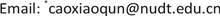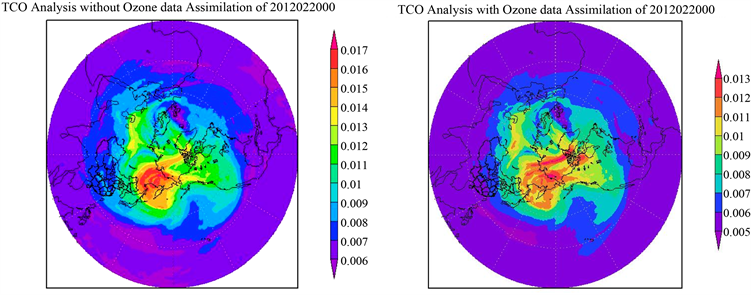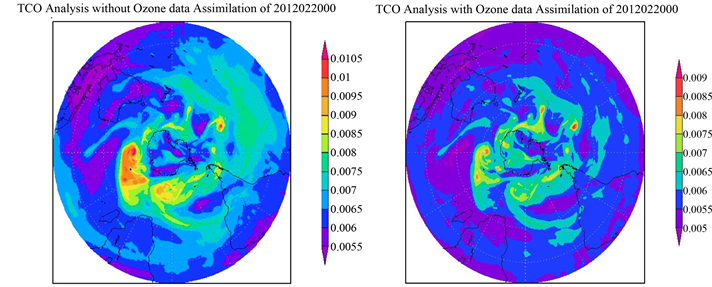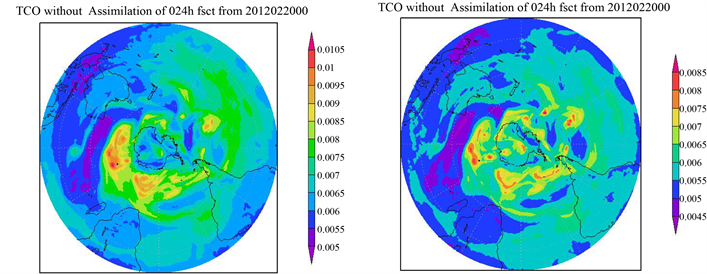﻿ 臭氧观测的全球四维变分资料同化技术 The Technologies for Global Four-Dimensional Variational Data Assimilation of Ozone Observations

Advances in Geosciences
Vol.07 No.06(2017), Article ID:23279,11 pages
10.12677/AG.2017.76088

The Technologies for Global Four-Dimensional Variational Data Assimilation of Ozone Observations

Xiaoqun Cao*, Junqiang Song, Jun Zhao, Hongze Leng, Shuo Ma

College of Meteorology and Oceanology, National University of Defense Technology, Changsha HunanReceived: Dec. 6th, 2017; accepted: Dec. 21st, 2017; published: Dec. 29th, 2017ABSTRACT

In order to solve the problems of being short of stratospheric atmospheric observations, a method of improving the global numerical weather prediction (NWP) qualities is proposed by assimilating satellite ozone data. The complex problem of ozone data assimilation is transformed into a large-scale optimization problem constrained by the governing equations of atmospheric motion, and the global four-dimensional variational data assimilation of ozone from SCIAMACHY remote sensor is implemented to produce initial fields for global NWP model. The numerical experimental results show that the utilization rates of surface and sounding observations have been upgraded to a certain extent due to the introduction of satellite ozone data assimilation, and the distribution of the ozone prediction field changes obviously. Furthermore, the forecast skills in the northern and southern hemispheres are improved a lot by carrying out the statistical verification.

Keywords:Numerical Weather Prediction, Four-Dimensional Variational Data Assimilation, Satellite Ozone Observations, SCIAMACHY, Adjoint ModelCopyright © 2017 by authors and Hans Publishers Inc.

This work is licensed under the Creative Commons Attribution International License (CC BY).

http://creativecommons.org/licenses/by/4.0/1. 引言

2. 臭氧资料同化技术

2.1. 四维变分资料同化方法

$J\left({x}_{0}\right)=\frac{1}{2}{\left({x}_{0}-{x}_{b}\right)}^{\text{T}}{B}^{-1}\left({x}_{0}-{x}_{b}\right)+\frac{1}{2}\underset{i=0}{\overset{N}{\sum }}{\left\{{H}_{i}\left[{M}_{{t}_{0},{t}_{i}}\left({x}_{0}\right)\right]-{y}_{i}^{o}\right\}}^{\text{T}}{O}_{i}^{-1}\left\{{H}_{i}\left[{M}_{{t}_{0},{t}_{i}}\left({x}_{0}\right)\right]-{y}_{i}^{o}\right\}$ (1)

2.2. 臭氧观测算子的设计

$T{O}_{3,k}=\frac{1}{g}\underset{i=1}{\overset{k}{\sum }}{q}_{i}\left({p}_{i}-{p}_{i-1}\right)$ (2)

2.3. 臭氧化学输运模式的切线性/伴随模式

$\begin{array}{l}\frac{\partial {c}_{i}}{\partial t}+\nabla \left(v{c}_{i}\right)-\nabla \left(\rho K\nabla \frac{{c}_{i}}{\rho }\right)-{\sum }_{r=1}^{R}\left\{k\left(r\right)\left[{s}_{i}\left({r}_{+}\right)-{s}_{i}\left({r}_{-}\right)\right]{\prod }_{j=1}^{U}{c}_{j}^{{s}_{j}\left({r}_{-}\right)}\right\}\\ ={E}_{i}+{D}_{i}\end{array}$ (3)

$\begin{array}{l}-\frac{\partial \delta {c}_{i}^{*}}{\partial t}+v\nabla \delta {c}_{i}^{*}-\frac{1}{\rho }\nabla \left(\rho K\nabla \delta {c}_{i}^{*}\right)\\ +{\sum }_{r=1}^{R}\left\{k\left(r\right)\frac{{s}_{i}\left({r}_{-}\right)}{{c}_{i}}{\prod }_{j=1}^{U}{c}_{j}^{{s}_{j}\left({r}_{-}\right)}{\sum }_{n=1}^{U}\left[{s}_{n}\left({r}_{+}\right)-{s}_{n}\left({r}_{-}\right)\right]\delta {c}_{n}^{*}\right\}=0\end{array}$ (4)

2.4. 臭氧资料同化求解

3. 臭氧资料同化数值实验

3.1. 卫星臭氧观测数据描述

3.2. 观测资料同化结果分析Table 1. Impact of satellite ozone data assimilation on the number on two kinds of conventional observations which are assimilatedTable 2. Screening results of all kinds of observation used in the global 4D-Var system (statistics of 12-hour long assimilation window at 00 UTC on February 20, 2012)

3.3. 臭氧总含量分析场对比Figure 1. Analysis Fields of Total Column Ozone (TCO) for the Northern Hemisphere at 00 UTC on February 20, 2012. The left picture shows TCO distribution without assimilation of SCIAMACHY ozone observations, while the right one shows the results by assimilating ozone data.Figure 2. Analysis Fields of Total Column ozone for the Southern Hemisphere at 00 UTC on February 20, 2012. The difference of left and right graphs are same as in Figure 1.

3.4. 臭氧总含量预报场对比

3.5. 分析预报技巧比较Figure 3. 24 hours Forecast Fields of Total Column ozone for the Southern Hemisphere from 00 UTC on February 20, 2012. The difference of left and right graphs are same as in Figure 1.Figure 4. 48 hours Forecast Fields of Total Column ozone for the Southern Hemisphere from 00 UTC on February 20, 2012. The difference of left and right graphs are same as in Figure 1.

4. 结论

The Technologies for Global Four-Dimensional Variational Data Assimilation of Ozone Observations[J]. 地球科学前沿, 2017, 07(06): 856-866. http://dx.doi.org/10.12677/AG.2017.76088

1. 1. 王革丽, 吕达仁, 杨培才. 人类活动对大气臭氧层的影响[J]. 地球科学进展, 2009, 24(3): 331-337.

2. 2. 吕达仁, 陈洪滨. 平流层和中层大气研究的进展[J]. 大气科学, 2003, 27(4): 750-769.

3. 3. 施春华, 陈月娟, 郑彬, 等. 平流层臭氧季节变化的动力和光化学作用之比较[J]. 大气科学, 2010, 34(2): 399-406.

4. 4. Morcrette, J.J. (2003) Ozone-Radiation Interactions in the ECMWF Forecast System. Tech. Memo. 375, ECMWF, Reading, UK.

5. 5. Dethof, A. and Holm, E. (2004) Ozone Assimilation in the ERA-40 Reanalysis Project. Quarterly Journal of the Royal Meteorological Society, 131, 2851-2872. https://doi.org/10.1256/qj.03.196

6. 6. Bregman, A., Krol, M.C. and Teyssdre, H. (2001) Chemistry Transport Mode1 Compar-ison with Ozone Observations in the Midlatitude Lowermost Stratosphere. Journal of Geophysical Research, 106, 17479-17496. https://doi.org/10.1029/2000JD900752

7. 7. Dethof, A. (2003) Assimilation of Ozone Retrievals from the MIPAS Instrument on Board ENVISAT. Tech. Memo. 428, ECMWF, Reading, UK.

8. 8. Errera, Q. and Fonteyn, D. (2001) Four-Dimensional Variational Chemical Assimilation of CRISTA Stratospheric Measurements. Journal of Geophysical Research, 106 (D11), 12253-12265. https://doi.org/10.1029/2001JD900010

9. 9. Eskes, H.J., Van Velthoven, P.F.J. and Valks, P.J.M. (2003) Assimilation of GOME Total Ozone Satellite Observations into a Three Dimensional Tracer Transport Model. Quarterly Journal of the Royal Me-teorological Society, 129, 1663-1681. https://doi.org/10.1256/qj.02.14

10. 10. Eskes, H.J., van der A.R.J. and Brinksma, E.J. (2005) Retrieval and Validation of Ozone Columns Derived from Measurements of SCIAMACHY on Envisat. Atmospheric Chemistry and Physics, 4429-4475. https://doi.org/10.5194/acpd-5-4429-2005

11. 11. Geer, A.J., Lahoz, W.A. and Jackson, D.R. (2007) Evaluation of Linear Ozone Photochemistry Parametrizations in a Stratosphere-Troposphere Data Assimilation System. Atmospheric Chemistry and Physics, 7, 939-959. https://doi.org/10.5194/acp-7-939-2007

12. 12. 王自发, 谢付莹, 王喜全. 嵌套网格空气质量预报模式系统的发展与应用[J]. 大气科学, 2006, 30(5): 778-790.

13. 13. 张凯, 徐大海, 朱蓉. CAPPS多箱模式中光化学模式的嵌套与城市大气臭氧数值预报[J]. 应用气象学报, 2005, 16(1): 1-12.

14. 14. 张美根. 多尺度空气质量模式系统及其验证I: 模式系统介绍与气象要素模拟[J]. 大气科学, 2005, 29(5): 805-813.

15. 15. 张伟, 王自发, 安俊岭. 利用BP神经网络提高奥运实时预报系统预报效果[J]. 气候与环境研究, 2010, 15(5): 595-601.

16. 16. 唐晓, 王自发, 朱江. 北京地面臭氧的集合预报试验[J]. 气候与环境研究, 2010, 15(5): 677-684.

17. 17. Tang, X., Zhu, J. and Wang, Z.F. (2011) Improvement of Ozone Forecast over Beijing Based on Ensemble Kalman Filter with Simultaneous Adjustment of Initial Conditions and Emissions. Atmospheric Chemistry and Physics, 11, 12901-12916. https://doi.org/10.5194/acp-11-12901-2011

18. 18. 黄思训, 伍荣生. 大气科学中的数学物理问题[M]. 北京: 气象出版社, 2001.

19. 19. 邹晓蕾. 资料同化理论与应用(上册) [M]. 北京: 气象出版社, 2009.

20. 20. 曹小群, 黄思训, 杜华栋. 变分同化中水平误差函数的正交小波模拟新方法[J]. 物理学报, 2008, 57(3): 1984-1989.

21. 21. 张卫民, 曹小群, 宋君强. 以全球谱模式为约束的四维变分资料同化系统YH4DVAR的设计和实现[J]. 物理学报, 2012, 61(24): 249202-13.

22. 22. 曹小群, 皇群博, 刘柏年. 基于对偶数理论的资料同化新方法[J]. 物理学报, 2015, 64(13): 130502-12.

23. 23. 曹小群, 宋君强, 张卫民. 一种基于复数域微分的资料同化新方法[J]. 物理学报, 2013, 62(17): 170504-9.

24. NOTES



*通讯作者。